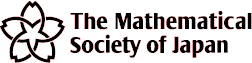MSJ Spring Meeting 2013
Venue: Yoshida-South Campus, Kyoto University
Dates: March 20-23, 2013

## List of Key Words

• Foundation of Mathematics and History
• 1 Foundation of Mathematics
• 2 History
• Complex Analysis
• 1 Function theory of one variable
• 2 Function theory of several variables
• Real Analysis
• 1 Fourier Analysis
• 2 Function Spaces
• 3 Evolution Equation
• 4 Geometry of Banach spaces
• 5 Measure theory and Theory of Integration
• 6 Other Topics
• Probability and Statistics
• 1 Probability Theory and Stochastic Process
• 2 Planning Mathematics
• 3 Probability Distributions, sample distributions, random digits
• 4 Experiment Design, 5 Multivariate Analysis
• 6 Time Series
• 7 Non- parametric Analysis
• 8 Asymptotic Theory
• 9 Application of Statistics
• 10 Theory of Inference (not included in 3-7)
• 11 Other Topics Applied Mathematics
• Applied Mathematics
• 1 Applied Mathematics using Analysis
• 2 Applied Mathematics related to Discrete Mathematics